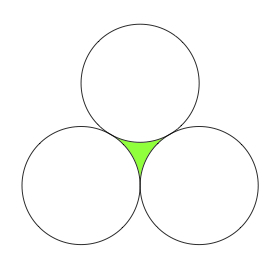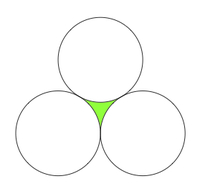# Mutually Tangent Circles

Alignments to Content Standards: G-C.B.5

Three circles, each having radius 2, are mutually tangent as pictured below:What is the total area of the circles together with the shaded region?## IM Commentary

This is a challenging task which requires students to carefully divide up the picture into different pieces for which the area is known. In particular, the area of the three circles is readily computed and so the key is to determine the shaded area. This can be done by noticing that when combined with three sectors of the circles it forms an equilateral triangle. Thus, in addition to understanding how to calculate the area of a circle sector, students will need to know the formula for the area of an equilateral triangle. The teacher may wish to either provide the picture in the solution or be willing to support students as they look for a means of calculating the shaded area. Even with the picture, much remains to be done particularly if students are requested to calculate the area of the equilateral triangle (an argument can be found here) or to justify carefully that this configuration of circles makes sense (more about this can be found here). Neither of these lines of thought is developed in the solution presented here.

This task was adapted from problem #16 on the 2012 American Mathematics Competition (AMC) 10B Test. As with many tasks from this source, a principal benefit is the opportunity to model practice standards alongside with content standards. While students do make use of their knowledge of areas of sectors of circles, more prominent is the requirement that students illustrate perseverance in solving problems and look for structure (Standards for Mathematical Practice 1 and 7, respectively).

For this test, taken by 35,086 students, the multiple choice answers for the problem had the following distribution:

 Choice Answer Percentage of Answers (A)* ${10\pi+4\sqrt{3}}$ 42.79 (B) ${13\pi - \sqrt{3}}$ 3.55 (C) ${12\pi +\sqrt{3}}$ 28.84 (D) ${10\pi + 9}$ 1.44 (E) ${13\pi}$ 1.41 Omit -- 21.95

Of the 35,086 students: 17,169, or 49%, were in 10th grade; 9,928 or 28%, were in 9th grade; and the remainder were below than 9th grade.

This task includes an experimental GeoGebra worksheet, with the intent that instructors might use it to more interactively demonstrate the relevant content material. The file should be considered a draft version, and feedback on it in the comment section is highly encouraged, both in terms of suggestions for improvement and for ideas on using it effectively. The file can be run via the free online application GeoGebra, or run locally if GeoGebra has been installed.

## Attached Resources

• Geogebra File
• ## Solution

The shaded shape is highly irregular with three different arcs of circles as its boundary. The key to calculating the area is to add into the picture the sectors of the three circles swept out by those arcs:The vertices of the blue shape are the centers of the three circles. Since each pair of circles is tangent, the centers of the circles are all 4 units apart. The sides of the blue shape are each made up out of two circle radii each measuring 2 units. This means that these radii are collinear and so the blue shape is a triangle. It is equilateral because each side has length 4 units. The area of an equilateral triangle with side length 4 units is 4$\times \sqrt{3}$ units$^2$.

The blue sectors are each 60 degrees since these are angles in an equilateral triangle. Together they form a 180 degree sector or half of a circle of radius 2. So the area of the green section is $$4 \sqrt{3} - 2 \pi \,\, \text{units}^2.$$ Each circle has area 4$\pi$ units$^2$. So this means that the sum of the area of the three circles and the green shaded portion is $10 \pi + 4 \sqrt{3}$.